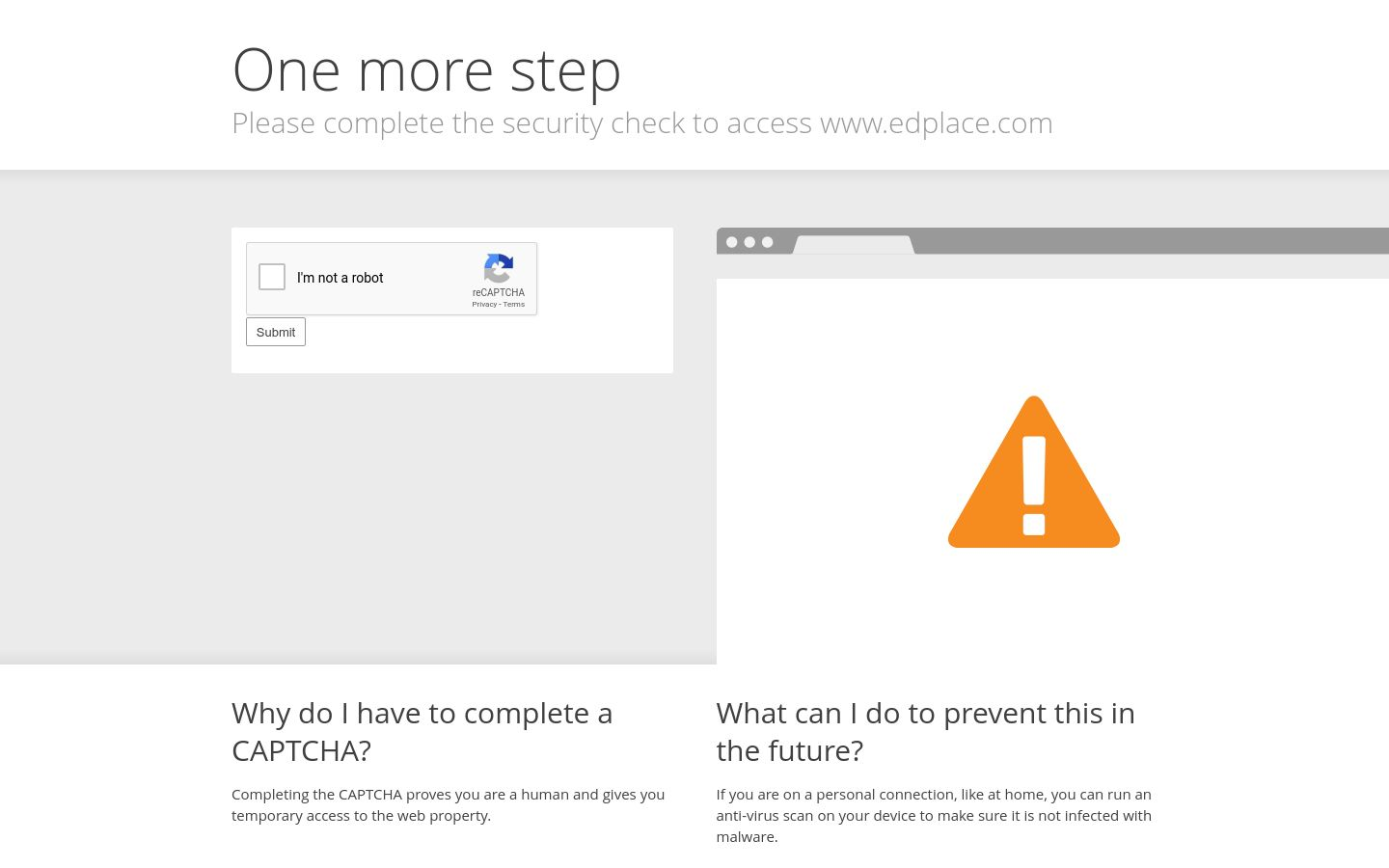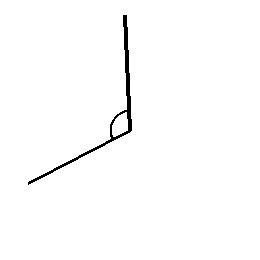# Geometry: Estimate Angles

In this worksheet, students use their knowledge to estimate an angle. Then they measure the angle to see how close they were.This content is premium and exclusive to EdPlace subscribers.Key stage:  KS 2

Curriculum topic:   Maths and Numerical Reasoning

Curriculum subtopic:   2D Shapes: Circles, Angles and Bearings

Difficulty level:#### Worksheet Overview

In this worksheet, we will learn how to estimate the size of each angle.

Question:

Estimate the size of the angle.Think of key angles such as 30°, 60°, 90°, 120° and 180°